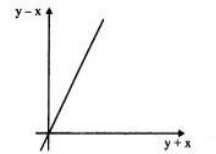Question 15

# The graph of y - x (on the y axis) against y + x (on the x axis) is as shown below. (All graphs in this question are drawn to scale and the same scale and the same scale has been used on each axis.)Which of the following shows the graph of y against x?

Solution

For a normal graph with y and x-axis, the equation of the line passing through the origin is y =mx where m is the slope of the line.

m is +ve if the angle made by the line with the x-axis is < $$90^{\circ\ }$$

$$\therefore\$$ The equation of the line in the given graph would be y-x = k( y+ x) since the axes are y-x and y+x and the line is passing through the origin.

k > 1 because the angle is greater than 45$$^{\circ\ }$$

$$y=\frac{k\left(x+1\right)}{1-k}$$

Since k>1

Therefore y<0 for x>-1 and y>0 for x<-1

Option d correctly satisfy this condition

• All Quant CAT Formulas and shortcuts PDF
• 30+ CAT previous papers with solutions PDF